## Isotomic Conjugate Point

The point of concurrenceof the Isotomic Lines relative to a point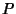. The isotomic conjugate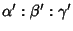of a point with Trilinear Coordinatesis(1)

The isotomic conjugate of a Linehaving trilinear equation(2)

is a Conic Section circumscribed on the Triangle(Casey 1893, Vandeghen 1965). The isotomic conjugate of the Line at Infinity having trilinear equation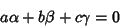(3)

is Steiner's Ellipse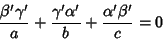(4)

(Vandeghen 1965). The type of Conic Section to whichis transformed is determined by whether the linemeets Steiner's Ellipse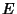.
1. Ifdoes not intersect, the isotomic transform is an Ellipse.

2. Ifis tangent to, the transform is a Parabola.

3. Ifcuts, the transform is a Hyperbola, which is a Rectangular Hyperbola if the line passes through the isotomic conjugate of the Orthocenter
(Casey 1893, Vandeghen 1965).

There are four points which are isotomically self-conjugate: the Centroidand each of the points of intersection of lines through the Vertices Parallel to the opposite sides. The isotomic conjugate of the Euler Line is called Jerabek's Hyperbola (Casey 1893, Vandeghen 1965).

Vandeghen (1965) calls the transformation taking points to their isotomic conjugate points the Cevian Transform. The product of isotomic and Isogonal is a Collineation which transforms the sides of a Triangle to themselves (Vandeghen 1965).

See also Cevian Transform, Gergonne Point, Isogonal Conjugate, Jerabek's Hyperbola, Nagel Point, Steiner's Ellipse

References

Casey, J. A Treatise on the Analytical Geometry of the Point, Line, Circle, and Conic Sections, Containing an Account of Its Most Recent Extensions with Numerous Examples, 2nd rev. enl. ed. Dublin: Hodges, Figgis, & Co., 1893.

Eddy, R. H. and Fritsch, R. The Conics of Ludwig Kiepert: A Comprehensive Lesson in the Geometry of the Triangle.'' Math. Mag. 67, 188-205, 1994.

Johnson, R. A. Modern Geometry: An Elementary Treatise on the Geometry of the Triangle and the Circle. Boston, MA: Houghton Mifflin, pp. 157-159, 1929.

Vandeghen, A. Some Remarks on the Isogonal and Cevian Transforms. Alignments of Remarkable Points of a Triangle.'' Amer. Math. Monthly 72, 1091-1094, 1965.